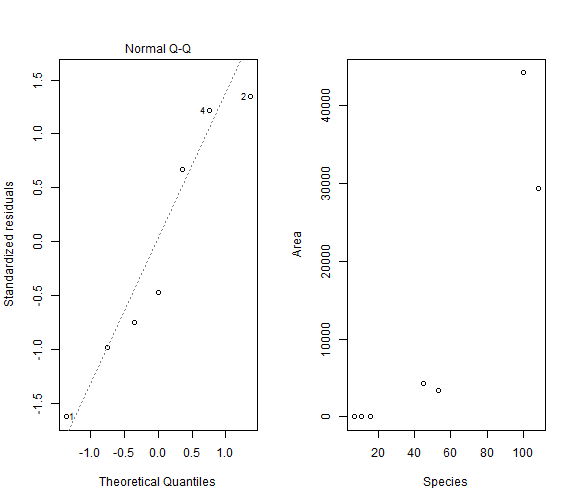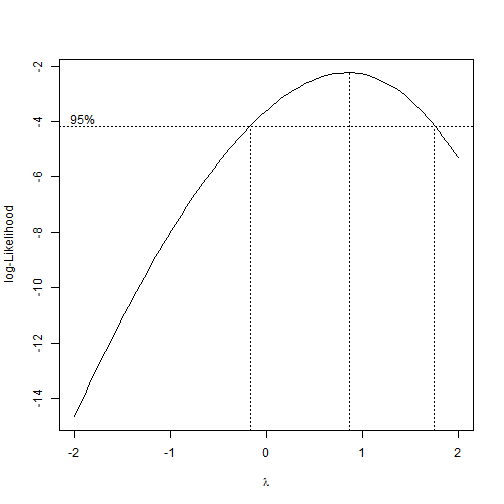# Box-Cox Transformation

A transformation which is very useful in applied linear regression problems is the Box-Cox transformation which was devised by Box & Cox (1964) to reduce non-normality, heteroscedasticity and non-linearity in the response variable. It is a power transformation of the form:

$y_i^{(\lambda)} = \begin{cases} \frac{y_{i}^{\lambda}-1}{\lambda} & \text{if } \lambda eq0\\ \log\left(\lambda\right) & \text{if }\lambda=0 \end{cases}$

When fitting a linear model, the assumed relationship can then be expressed as follows:

$Y^{(\lambda)} = X \beta + \epsilon$

As we can see, the transformed data do not cover the whole real numbers but are constrained (e.g. the $\log$). It follows that the transformed variables can never be exactly normally distributed, but that this can only be an approximation. A literature review about the Box-Cox transform pointing also to sources which discuss possible methods to estimat $$\lambda$$ is given by Sakia (1992).

In the following, we will use R to estimate $$\lambda$$ and apply the Box-Cox transform on a real data set.

We start by showing an example where the Box-Cox transform is not very helpful. Load the data from R package Sleuth3 which accompanies the excellent book “The Statistical Sleuth” by Ramsey and Schafer.

library(Sleuth3)
data(case0801)
attach(case0801)


The data set is

library(xtable)
print(xtable(case0801), type = \"html\")


AreaSpecies
1 44218 100
2 29371 108
3 4244 45
4 3435 53
5 32 16
6 5 11
7 1 7

Fitting the model by calling

lm1 = lm(Species ~ Area, data = case0801)


As can be seen from the quantile-quantile plot depicted next, we might face violation of the normality assumption, although it is quite hard to tell given the amount of data:

par(mfrow = c(1, 2))
plot(lm1, which = c(2))
plot(Area ~ Species)In addition, the plot on the right-hand side suggests that the relationship might be non-linear. We now use the Box-Cox transformation in order to decide an appropriate transformation

library(MASS)
boxcox(lm1)In the figure, a 95% confidence interval for $$\lambda$$ are displayed. As we can see, the range of this interval is very large, suggesting that we cannot choose an approrpiate transformation based on this output. This occurs frequently in situations with so few data points.